• Save

# Pie Chart Definition and Problems Solutions

8 months ago 712 Views
NEW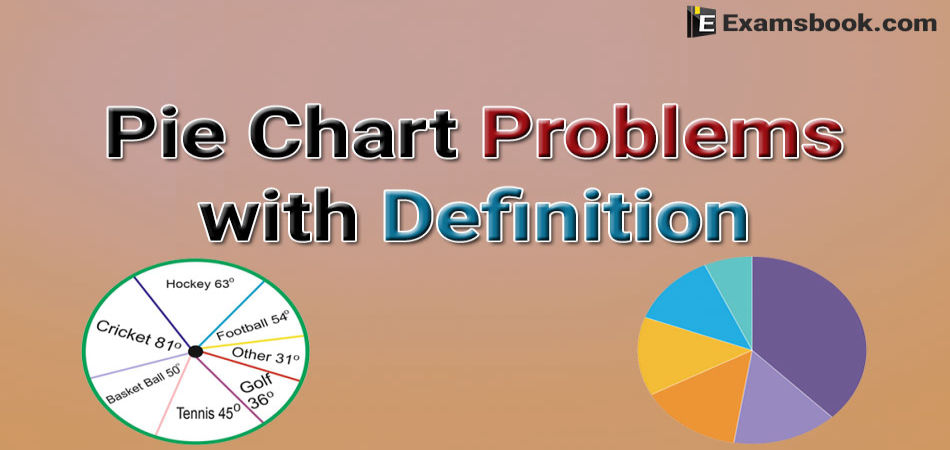If you are preparing for any competitive exams like SSC, Bank, Railway, etc. this pie chart blog will be very helpful for you. So, you should practice these Pie Chart Problems Solutions to get good marks in your competitive exams with Pie Chart Definition.

A pie chart (or a circle chart) is a circular statistical graphic, which is divided into slices to represent the numerical proportion. In a pie chart, the arc length of each slice (and consequently its central angle and area), is proportional to the quantity it represents.

So you can easily practice by these pie chart problems with examples and know more about Pie Chart Formulas with Explanation for your better performance.

## Pie Chart Problems with Solutions

Direction: The circle-graph given here shows the spending of a country on various sports during a particular year. Study the graph carefully and answer the question given below it.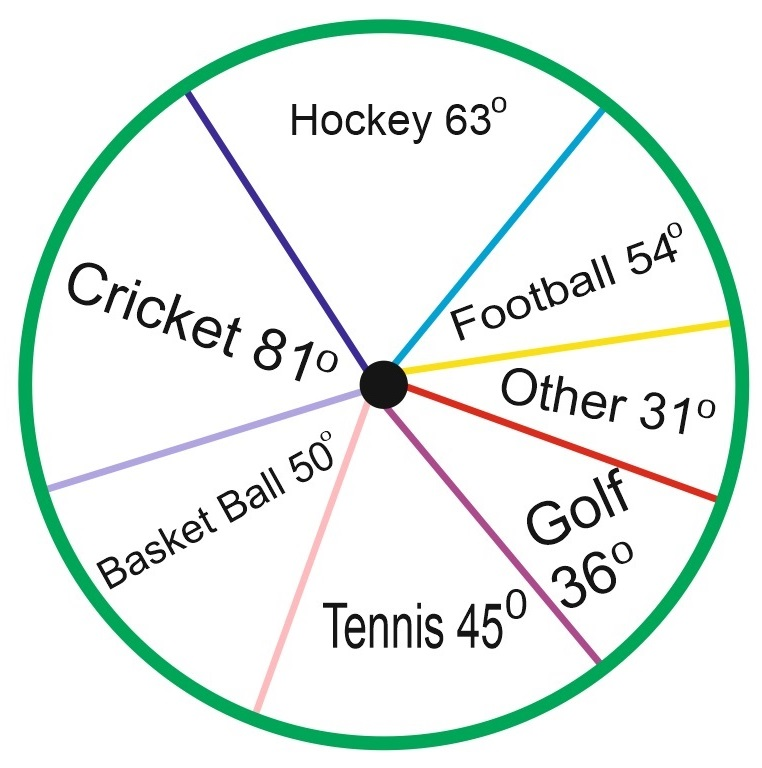Q.1. What percent of the total spending is spent on Tennis?

(A)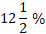(B)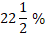(C) 25 %

(D) 45 %

Ans .  A

Percentage of money spent on Tennis =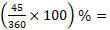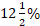Direction (Question 2 to 10): The following pie-chart shows the percentage distribution of the expenditure incurred in published a book. Study the pie-chart and answer the question based on it.

VARIOUS EXPENDITURES (IN PERCENTAGE) INCURRED IN PUBLISHING A BOOK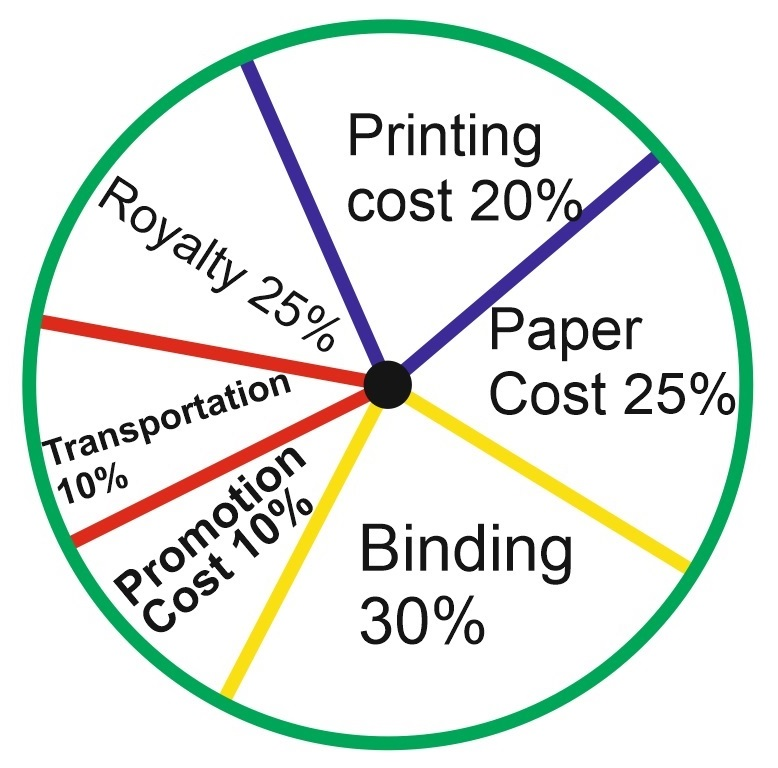Q.2. If for a certain quantity of books, the publisher has to pay Rs. 30,600 as printing cost, then what will be the amount of royalty to be paid for these books?

(A) Rs. 19,450

(B) Rs. 21,200

(C) Rs. 22,950

(D) Rs. 26,150

Ans .   C

5. Let the amount of Royalty to be paid for these books be Rs. p.

Then, 20 : 15 = 30600 : r ⇒ r = Rs.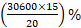= Rs. 22950.

Q.3. The price of the book is marked 20% above the C.P. If the market price of the book is Rs. 180, then what is the cost of the paper used in a single copy of the books?

(A) Rs. 36

(B) Rs. 37.50

(C) Rs. 42

(D) Rs. 44.25

Ans .   B

6. Clearly, marked price of the book = 120% of C.P

Also, cost of paper = 25% of C.P.

Let the cost of paper for a single book be Rs. n.

Then, 120 : 25 = 180 : n ⇒ n = Rs.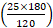= Rs. 37.50

Q.4. What is the central angle of the sector corresponding to the expenditure incurred on Royalty?

(A) 150

(B) 240

(C) 540

(D) 480

Ans .   C

1. Central angle corresponding to Royalty = (15% of 360)= 540

Q.5. For an edition of 12,500 copies, the amount of Royalty paid by the publisher is Rs. 2,18,250. What should be the selling price of the book if the publisher desires a profit of 5%?

(A) Rs. 152.50

(B) Rs. 157.50

(C) Rs. 162.50

(D) Rs. 167.50

Ans .   B

7. Clearly, S.P. of the book = 105% of C.P.

Let the selling price of this edition (of 12500 books) be Rs. x.

Then, 15 : 105 = 281250 : x ⇒ x = Rs.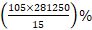= Rs. 1968750.

⸫ S.P of one book = Rs.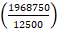= Rs. 157.50

Q.6. Which two expenditures together have a central angle of 1080?

(A) Binding Cost and Transportation Cost

(B) Printing Cost and Paper Cost

(C) Royalty and Promotion Cost

(D) Binding Cost and Paper Cost

Ans .   A

2. Central angle of 1080 =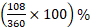of the total expenditure

= 30% of the total expenditure.

From the pie – chart it is clear that:

Binding Cost + Transportation Cost

= (20% + 10%) of the total expenditure

= 30% of the total expenditure

⸫ Binding Cost and Transportation Cost together have a central angle of 1080.

Q.7. If 5500 copies are published and the transportation cost on them amounts to Rs. 82,500, then what should be the selling price of the book so that the publisher can earn a profit of 25%?

(A) Rs. 187.50

(B) Rs. 191.50

(C) Rs. 175

(D) Rs. 180

Ans .   A

8. For the publisher to earn a profit of 25% S.P = 125% of C.P.

Also Transportation Cost = 10% of C.P.

Let the S.P of 5500 books be Rs. x.

Then, 10 : 125 = 82500 : x ⇒ x = Rs.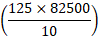= Rs. 1031250.

⸫ S.P. of one book = Rs.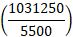= Rs. 187.50.

Q.8. If the difference between the two expenditures is represented by 180 in the pie-chart, then these expenditures possibly are:

(A) Binding Cost and Promotion Cost

(B) Paper Cost and Royalty

(C) Binding Cost and Printing Cost

(D) Paper Cost and Printing Cost

Ans .   D

3. Central angle of 180 =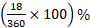of the total expenditure

= 5% of the total expenditure.

From the pie-chart it is clear that:

Out of the given combinations, only in combination (d) the difference is 5% i.e.

Paper Cost – Printing cost

= (25% - 20%) of the total expenditure

= 5% of total expenditure.

Q.9. Royalty on the book is less than the printing cost by :

(A) 5%

(B)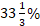(C) 20%

(D) 25%

Ans .   D

9. Printing Cost of book = 20% of C.P.

Royalty on book = 15% of C.P

Difference = (20% of C.P) – (15% of C.P) = 5% of C.P.

⸫ Percentage difference =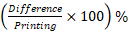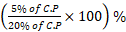= 25% = 25%

Thus, Royalty on the book is 25% less than the printing Cost.

Q.10. If for an edition of the book, the cost of paper is Rs. 56250, then find the promotion cost for this edition?

(A) Rs. 20,000

(B) Rs. 22,500

(C) Rs. 25,500

(D) Rs. 28,125

Ans .   B

4. Let the Promotion cost for this edition be Rs. p.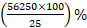Then, 25 : 10 = 56250 : p ⇒ p = Rs.= Rs. 22500.